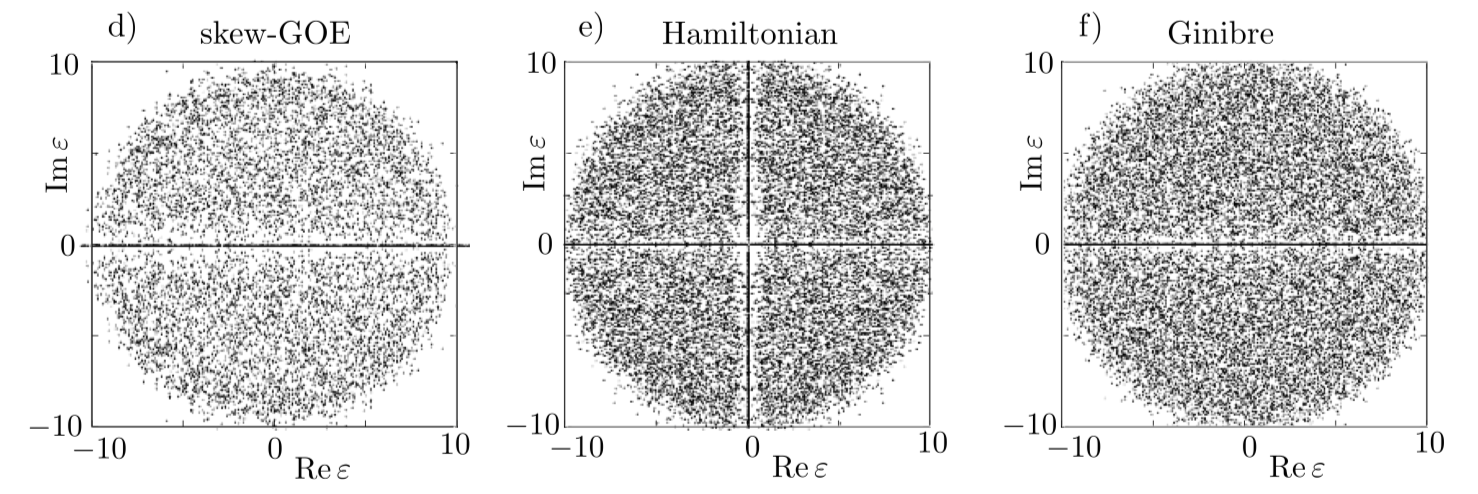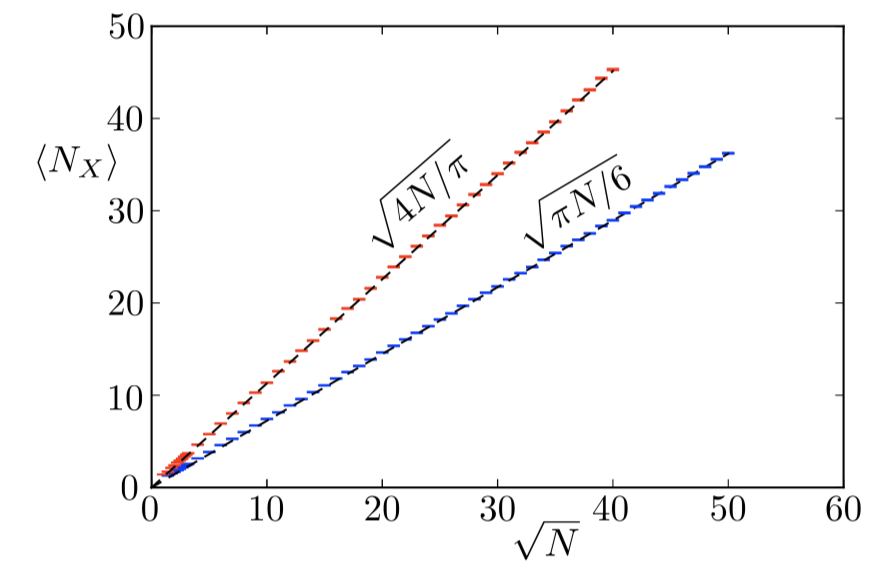# Random complex eigenvalues and averages of traces

I have asked this in MSE here, but got no interesting answers.

Suppose I have a random matrix $M$ of dimension $N$ which is real, but not symmetric. Suppose I know that, for large $N$, the marginal distribution of its eigenvalues is uniform over the unit disk in the complex plane. What does that tell me about the average values of traces, $\langle {\rm Tr}(M^n)\rangle$?

Of course $\langle {\rm Tr}(M^n)\rangle \to 0$ as $N\to\infty$, but can I know the asymptotics?

Does it follow from the "disk law" for the eigenvalues that the singular values have a quarter-circle distribution?

Summary: I don't think the average $\langle {\rm Tr}(M^n)\rangle \to 0$ for $N\rightarrow\infty$, at least for $n$ even I will argue this average is $\propto\sqrt N$ because of the contributions from eigenvalues on the real axis.

• Consider first the eigenvalues $\lambda_p=r_p e^{i\phi_p}$ of $M$ off the real axis. Because $M$ is real these must come in complex conjugate pairs, $\lambda_p=\lambda^\ast_{N-p}$, so $${\rm Tr}\, M^n=2\sum_{p=1}^{N/2} r_p^n \cos n\phi_p,$$ and the average $$\langle{\rm Tr}\,M^n\rangle_{\rm complex}=N\int_0^\infty dr \int_0^{2\pi}d\phi\, P(r,\phi)\, r^{n+1} \cos n\phi.$$ Under the assumption that the eigenvalue density $P(r,\phi)$ in the complex plane is rotationally invariant in the unit disc this average is zero.

• Now turn to the eigenvalues on the real axis. There are $N_{\rm real}=c\sqrt N$ real eigenvalues for $N\gg 1$, where the coefficient $c$ depends on the distribution of $M$. (For a Gaussian $M$ one has $c=\sqrt{2/\pi}$.) The real eigenvalues are uniformly distributed in the interval $(-1,1)$. So their contribution to the average is $$\langle {\rm Tr}\,M^n\rangle_{\rm real}=c\sqrt{N}\int_{-1}^{1} r^n dr=\begin{cases} \frac{2c}{n+1}\sqrt{N}&\text{if n is even},\\ 0&\text{if n is odd}. \end{cases}$$

It is likely that the deviations from uniformity in the distribution of complex eigenvalues also give a nonzero contribution of the same order $\sqrt N$ as those from the real axis. (Basically because the eigenvalues that are pinned to the real axis repel nearby eigenvalues with nonzero imaginary part, see plot below.)Illustration of the eigenvalue distribution in the complex plane for three different ensembles of real random matrices (of size $N=100$). You can see the eigenvalues pinned to the real axis (they appear as a horizontal black line of dense points), surrounded by a depletion zone (white region of few points). The ensemble of Hamiltonian matrices has an additional symmetry that also pins eigenvalues to the imaginary axis. In each case the number $N_X$ of real eigenvalues scales as $\sqrt N$, with a different numerical prefactor (red line for Hamiltonian ensemble, blue for skew-GOE, Ginibre has $\sqrt{2N/\pi}$).
Figures from arxiv:1305.2924.

• In the last part of your answer, you are actually assuming that the distribution of eigenvalues is not uniform over the disc, right? I know that is the case for real Ginibre ensemble, so I guess you are assuming that this is universal... – thedude Jul 24 '18 at 22:31
• indeed, the $\sqrt N$ law is generic, I added a plot and a reference to illustrate this; for a uniform distribution you will need complex matrices, rather than real matrices. – Carlo Beenakker Jul 25 '18 at 6:17
• Dear Carlo, are you aware of published results for the traces of powers for real Ginibre? – thedude Nov 3 '18 at 0:11
• Another point. The sum over complex eigenvalues $\sum_\lambda \lambda^n$ could in principle also be of order $\sqrt{N}$, right? – thedude Nov 3 '18 at 0:12
• yes, all eigenvalues within the unit circle that lie in a strip of width $1/\sqrt N$ around the real axis will contribute order $\sqrt N$ to the sum. – Carlo Beenakker Nov 3 '18 at 7:41

Carlo's answer addresses the first question. To address the second one: the "disk law" (better known as the "circular law") does not tell you the distribution of singular values. However, the Marchenko-Pastur law does, and it is true that the singular values have a quarter-circle distribution, but with a different normalization than the circular law would lead you to expect.

The following only addresses the last question on the relation between eigenvalues and singular values.

What the eigenvalue distribution does not tell you, are traces in products of $M$ and $M^*$; since the singular values are given by the distribution of $MM^*$, the eigenvalue distribution does not contain enough information to conclude from this on the singular values. For example, you can compare the limit of the Ginibre ensemble and a normal random matrix ensemble (i.e., one with $MM^*=M^*M$) which has asymptotically a uniform distribution on the disk. So both ensembles have the disk law for the eigenvalue distribution, but in the first case the singular values have the quarter-circular distribution, whereas in the second case one gets, I think, a linear density for the singular values.